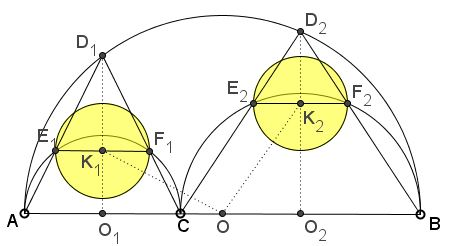A Newly Born Pair of Siblings to Archimedes' Twins: What is this about? A Mathematical Droodle

DiscussionArbelos is a geometric shape bounded by three semicircles. Arbelos was researched yet by Archimedes in his Book of Lemmas. In particular, Archimedes discovered two equal circles inscribed into arbelos that, since then, have been known as Archimedes' twins. In 1974, L. Bankoff found a sibling of the same size. The family has been expanded by C. W. Dodge and coauthors in 1999 and additional quadruplets have been delivered by F. Power in 2005.

Bùi Quang Tuån came up (November 12, 2011) with another pair.

An arbelos is comprised of three semicircles $(O_{1}),$ $(O_{2}),$ $O,$ with points of tangency $A,$ $C,$ $B.$ Let $r_{1},$ $r_{2}$ be the radii of $(O_{1}),$ $(O_{2}),$ respectively. Perpendicular bisector of diameter $AC$ intersects semicircle $(O)$ at $D_{1}.$ $D_{1}A$ and $D_{1}C$ intersect the semicircle $(O_{1})$ at $E_{1},$ $F_{1},$ respectively. Denote by $(K_{1})$ the circle with $E_{1}F_{1}$ as a diameter. Construct similarly $D_{2},$ $E_{2},$ $F_{2},$ $(K_{2}).$ Then1. Two circles $(K_{1}),$ $(K_{2})$ are congruent with Archimedes' twin circles i.e their radius: $\displaystyle r = \frac{r_{1}\cdot r_{2}}{r_{1} + r_{2}}.$

2. Their centers $K_{1}, K_{2}$ are equidistant from the center $O$ of the big circle: $K_{1}O = K_{2}O.$

Proof

To prove the first assertion, observe that (because of the symmetry in $O_{1}D_{1})$ $E_{1}F_{1}||AC.$ Draw $CE_{1}$ and $BD_{1}.$ Both are perpendicular to $AD_{1}$ and are, therefore, also parallel. We have several proportions:

$E_{1}F_{1}/AC = D_{1}E_{1}/AD_{1} = BC/AB = 2\cdot r_{2}/(2\cdot r_{1} + 2\cdot r_{2}) = r_{2}/(r_{1} + r_{2}).$

But $AC = 2r_{1},$ so that$E_{1}F_{1} = 2r_{1}r_{2}/(r_{1} + r_{2}),$

half of which is exactly the radius of Archimedes' twins. The expression is symmetric in $r_{1}$ and $r_{2},$ meaning that a similar derivation for the length of $E_{2}F_{2}$ will give the same result.

For the second assertion, apply the Pythagorean theorem to, say, $\Delta OO_{1}K_{1}.$ Indeed, we can find the legs $K_{1}O_{1}$ and $O_{1}O$ in terms of $r_{1}$ and $r_{2}.$

$K_{1}O_{1}/D_{1}O_{1} = AE_{1}/AD_{1} = AC/AB = 2\cdot r_{1}/(2\cdot (r_{1} + r_{2})) = r_{1}/(r_{1} + r_{2})\\ D_{1}O_{1}^{2} = AO_{1}\cdot BO_{1} = r_{1}\cdot (r_{1} + 2\cdot r_{2})\\ K_{1}O_{1}^{2} = r_{1}\cdot (r_{1} + 2\cdot r_{2})\cdot ( r_{1}/(r_{1} + r_{2}))^{2}.$

Now, $O_{1}O_{2} = r_{1} + r_{2};$ it follows that $O_{1}O = r_{2}.$ The Pythagorean theorem - $K_{1}O^{2} = K_{1}O_{1}^{2} + O_{1}O^{2}$ - then yields

$K_{1}O^{2} = (r_{1} + r_{2})^{2} - r_{1}\cdot r_{2}\cdot (2\cdot r_{1} + r_{2})\cdot (r_{1} + 2\cdot r_{2})/(r_{1} + r_{2})^{2}.$

This expression is again symmetric in $r_{1}$ and $r_{2},$ so that $K_{2}O^{2}$ evaluates to the same quantity: $K_{1}O = K_{2}O.$

An Extra Property of the Configuration

Bùi Quang Tuån has established an additional property of the configuration.

Define $G_{1}=E_{1}D_{1}\cap (K_{1}),$ $H_{1}=F_{1}D_{1}\cap (K_{1}),$ $G_{2}=E_{2}D_{2}\cap (K_{2}),$ $H_{2}=F_{2}D_{2}\cap (K_{2}).$Then $G_{1}H_{1}+G_{2}H_{2}=2r.$

Note that the shape that consists of the semicircle $(O_{1})$ and $\Delta AD_{1}C$ is similar to the figure that consists of the upper semicircle $(K_{1})$ and $\Delta E_{1}D_{1}F_{1},$ such that chord $E_{1}F_{1}$ in $(O_{1})$ corresponds to the chord $G_{1}H_{1}$ in $(K_{1}).$ This implies a proportion:

$\displaystyle\frac{G_{1}H_{1}}{E_{1}F_{1}}=\frac{E_{1}F_{1}}{AC}=\frac{r}{r_{1}},$

from which $\displaystyle G_{1}H_{1}=\frac{2r^{2}}{r_{1}}=\frac{2r_{1}r_{2}^{2}}{(r_{1}+r_{2})^{2}}.$ Similarly, $\displaystyle G_{2}H_{2}=\frac{2r_{1}^{2}r_{2}}{(r_{1}+r_{2})^{2}}.$ Adding the two gives

$\displaystyle G_{1}H_{1}+G_{2}H_{2}=\frac{2r_{1}r_{2}(r_{1}+r_{2})}{(r_{1}+r_{2})^{2}}=\frac{2r_{1}r_{2}}{(r_{1}+r_{2})}=2r.$